# Simplification Of Boolean Functions

## Simplification Using Algebraic Functions

In this approach, one Boolean expression is minimized into an equivalent expression by applying Boolean identities.

### Problem 1

Minimize the following Boolean expression using Boolean identities −

$$F (A, B, C) = A'B + BC'+ BC + AB'C'$$

### Solution

Given,$F (A, B, C) = A'B + BC'+ BC + AB'C'$

Or,$F (A, B, C) = A'B + (BC'+ BC') + BC+ AB'C'$

[By idempotent law, BC’ = BC’ + BC’]

Or,$F (A, B, C) = A'B + (BC'+ BC) + (BC'+ AB'C')$

Or,$F (A, B, C) = A'B + B(C'+ C) + C'(B+ AB')$

[By distributive laws]

Or,$F (A, B, C) = A'B + B.1 + C'(B + A)$

[ (C' + C) = 1 and absorption law (B + AB')= (B + A)]

Or,$F (A, B, C) = A'B + B + C'(B + A)$

[ B.1 = B ]

Or,$F (A, B, C) = B(A'+ 1) + C'(B + A)$

Or,$F (A, B, C) = B.1 + C'(B + A)$

[ (A' + 1) = 1 ]

Or,$F (A, B, C) = B + C'(B + A)$

[ As, B.1 = B ]

Or,$F (A, B, C) = B + BC' + AC'$

Or,$F (A, B, C) = B(1 + C') + AC'$

Or,$F (A, B, C) = B.1 + AC'$

[As, (1 + C') = 1]

Or,$F (A, B, C) = B + AC'$

[As, B.1 = B]

So,$F (A, B, C) = B + AC'$is the minimized form.

### Problem 2

Minimize the following Boolean expression using Boolean identities −

$$F (A, B, C) = (A + B) (A + C)$$

### Solution

Given, $F (A, B, C) = (A + B) (A + C)$

Or, $F (A, B, C) = A.A + A.C + B.A + B.C$ [Applying distributive Rule]

Or, $F (A, B, C) = A + A.C + B.A + B.C$ [Applying Idempotent Law]

Or, $F (A, B, C) = A(1 + C) + B.A + B.C$ [Applying distributive Law]

Or, $F (A, B, C) = A + B.A + B.C$ [Applying dominance Law]

Or, $F (A, B, C) = (A + 1).A + B.C$ [Applying distributive Law]

Or, $F (A, B, C) = 1.A + B.C$ [Applying dominance Law]

Or, $F (A, B, C) = A + B.C$ [Applying dominance Law]

So, $F (A, B, C) = A + BC$ is the minimized form.

## Karnaugh Maps

The Karnaugh map (K–map), introduced by Maurice Karnaughin in 1953, is a grid-like representation of a truth table which is used to simplify boolean algebra expressions. A Karnaugh map has zero and one entries at different positions. It provides grouping together Boolean expressions with common factors and eliminates unwanted variables from the expression. In a K-map, crossing a vertical or horizontal cell boundary is always a change of only one variable.

### Example 1

An arbitrary truth table is taken below −

A B A operation B
0 0 w
0 1 x
1 0 y
1 1 z

Now we will make a k-map for the above truth table −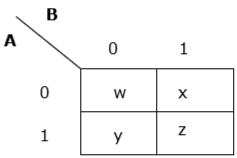### Example 2

Now we will make a K-map for the expression − AB+ A’B’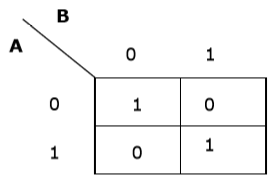## Simplification Using K-map

K-map uses some rules for the simplification of Boolean expressions by combining together adjacent cells into single term. The rules are described below −

Rule 1 − Any cell containing a zero cannot be grouped.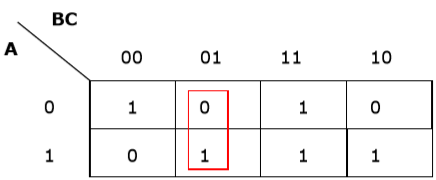Wrong grouping

Rule 2 − Groups must contain 2n cells (n starting from 1).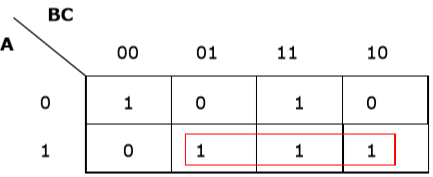Wrong grouping

Rule 3 − Grouping must be horizontal or vertical, but must not be diagonal.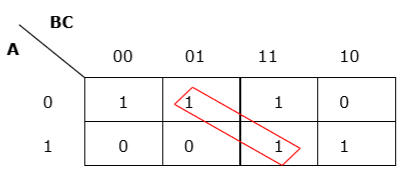Wrong diagonal grouping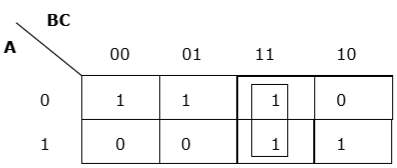Proper vertical grouping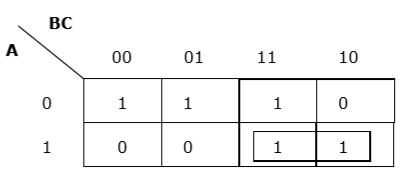Proper horizontal grouping

Rule 4 − Groups must be covered as largely as possible.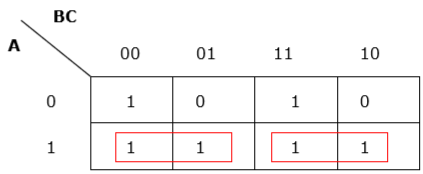Insufficient grouping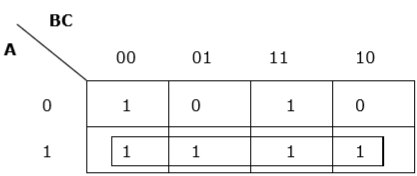Proper grouping

Rule 5 − If 1 of any cell cannot be grouped with any other cell, it will act as a group itself.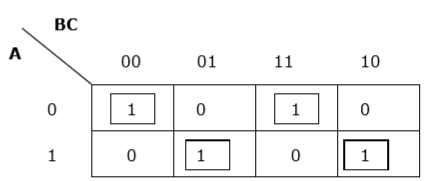Proper grouping

Rule 6 − Groups may overlap but there should be as few groups as possible.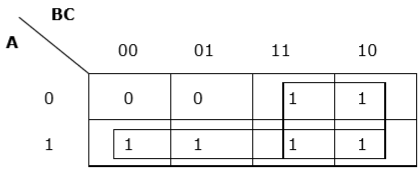Proper grouping

Rule 7 − The leftmost cell/cells can be grouped with the rightmost cell/cells and the topmost cell/cells can be grouped with the bottommost cell/cells.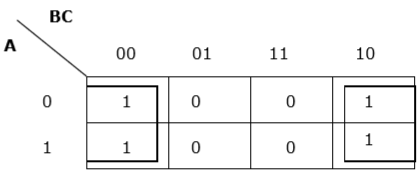Proper grouping

### Problem

Minimize the following Boolean expression using K-map −

$$F (A, B, C) = A'BC + A'BC' + AB'C'+ AB'C$$

### Solution

Each term is put into k-map and we get the following −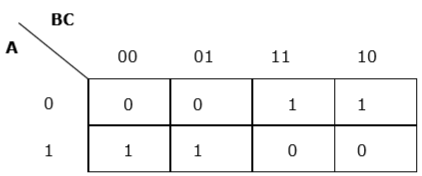K-map for F (A, B, C)

Now we will group the cells of 1 according to the rules stated above −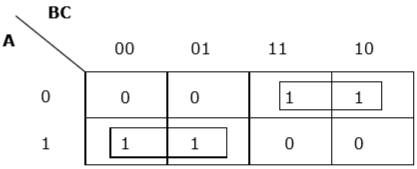K-map for F (A, B, C)

We have got two groups which are termed as $A’B$ and $AB’$. Hence, $F (A, B, C) = A’B+ AB’= A \oplus B$. It is the minimized form.